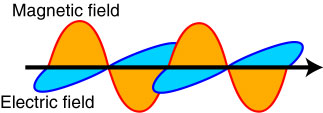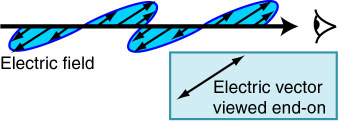# Polarization

This is a brief summary of polarization.Figure 1. An electromagnetic wave.

The intimate relationship between electric charges, magnetic fields and electromagnetic radiation was unravelled in the latter part of the 19th century by a number of physicists, not least James Clerk Maxwell who correctly deduced that light was an electromagnetic wave and thereby derived a value for the speed of light. According to Maxwell's description an electromagnetic wave consists of two transverse waves propagating in planes at right angles to one another, Figure 1. One wave is the electric field and the other the magnetic field. A transverse wave motion is one in which the amplitude of some physical property varies periodically in a direction perpendicular to that in which the wave is travelling. Waves on a plucked or flicked string are a good illustration of this - the string rises and falls as the wave travels along the string.

The wavelength is the distance between two corresponding parts of the wave e.g. the separation of adjacent troughs or adjacent peaks. If one were to sit at a point on the axis of the wave and watch it travel past, then the frequency of the wave is just the number of peaks (or troughs) which pass by each second. It is now measured in Hertz (units of 1/seconds, usually written as s-1) although it used to have the more descriptive unit of cycles per second.Figure 2. Linear polarisation.

The wave depicted in Figure 1 has an electric field that remains in the same plane throughout its motion. The way in which the electric field vector varies with time is shown as a set of arrows in Figure 2. If viewed into the oncoming beam of radiation i.e. looking from the right in this diagram then we would see the electric field vector increasing then decreasing in magnitude whilst oscillating in direction but always remaining horizontal. This is called a linearly polarised wave. As long as the electric field vector lies in a single plane then it is linearly polarised - it need not be horizontal, it can be at any angle. However, this is not always going to be the case. The electric field could have components which cause the electric field vector to rotate as viewed into the incoming beam. If the vector rotates in a clockwise direction the wave is said to have left-handed circular polarisation, if anti-clockwise then it is right-handed.

Naturally-generated electromagnetic waves are usually unpolarized, meaning that the direction changes randomly on the timescale of a few wave periods.